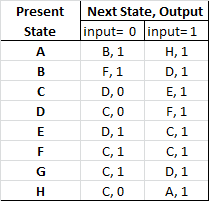# GATE | GATE CS 1997 | Question 69

Following is a state table for some finite state machine.A). Find the equivalence partition on the states of the machine.
B). Give the state table for the minimal machine. (Use appropriate names for the equivalent states. For example if states X and Y are equivalent then use XY as the name for the equivalent state in the minimal machine.)